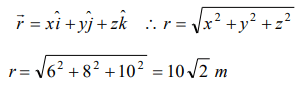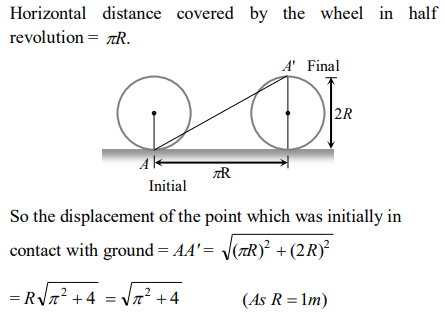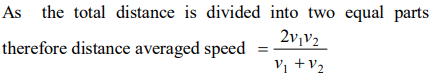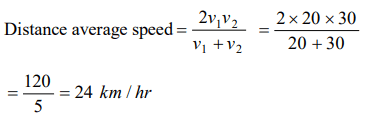## Motion in a Straight Line Questions and Answers Part-1

1. A Body moves 6 m north. 8 m east and 10m vertically upwards, what is its resultant displacement from initial position
a) $10\sqrt{2m}$
b) 10 m
c) $\frac{10}{\sqrt{2}}m$
d) $10\times 2m$

Explanation:2. A man goes 10m towards North, then 20m towards east then displacement is
a) 22.5m
b) 25m
c) 25.5m
d) 30m

Explanation:3. A person moves 30 m north and then 20 m towards east and finally $30\sqrt{2 }m$  in south-west direction. The displacement of the person from the origin will be
a) 10 m along north
b) 10 m long south
c) 10 m along west
d) Zero

Explanation:4. An aeroplane flies 400 m north and 300 m south and then flies 1200 m upwards then net displacement is
a) 1200 m
b) 1300 m
c) 1400 m
d) 1500 m

Explanation:5. An athlete completes one round of a circular track of radius R in 40 sec. What will be his displacement at the end of 2 min. 20 sec
a) Zero
b) 2R
c) $2\pi R$
d) $7\pi R$

Explanation: Total time of motion is 2 min 20 sec = 140 sec. As time period of circular motion is 40 sec so in 140 sec. athlete will complete 3.5 revolution i.e., He will be at diametrically opposite point i.e., Displacement = 2R.

6. A wheel of radius 1 meter rolls forward half a revolution on a horizontal ground. The magnitude of the displacement of the point of the wheel initially in contact with the ground is
a) $2\pi$
b) $\sqrt{2\pi}$
c) $\sqrt{\pi^{2}+4}$
d) $\pi$

Explanation:7. A person travels along a straight road for half the distance with velocity $v_{1}$ and the remaining half distance with velocity $v_{2}$ The average velocity is given by
a) $v_{1}v_{2}$
b) $\frac{V_2^2}{V_1^2}$
c) $\frac{v_{1}+v_{2}}{2}$
d) $\frac{2v_{1}v_{2}}{v_{1}+v_{2}}$

Explanation:8. The displacement-time graph for two particles A and B are straight lines inclined at angles of $30^{\circ}$ and $60^{\circ}$ with the time axis. The ratio of velocities of ${v_{A}:v_{B}}$  is
a) $1:2$
b) $1:\sqrt{3}$
c) $\sqrt{3}:1$
d) $1:3$

Explanation:9. A car travels from A to B at a speed of 20 km / hr and returns at a speed of 30 km / hr . The average speed of the car for the whole journey is
a) $25 km\diagup hr$
b) $24 km\diagup hr$
c) $50 km\diagup hr$
d) $5 km\diagup hr$